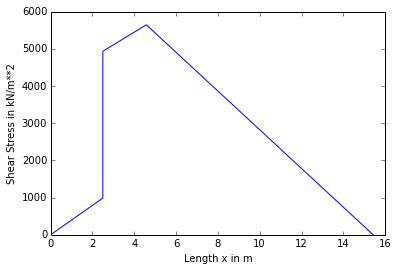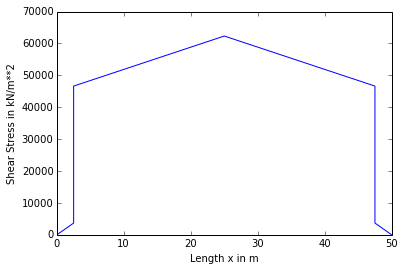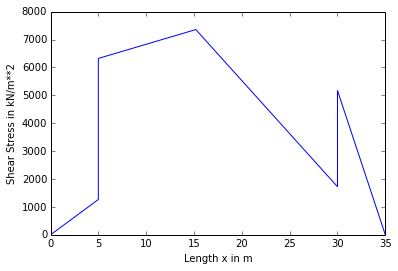# Chapter No.13:Shear Stress in Beams¶

## Problem no 13.2,Page No.301¶

In :
import math

#Initilization of variables
#W=10*w #KN/m #u.d.l
sigma=805*10**6 #Pa  #Bending stress
Tou=0.85*10**6 #Pa #Shear stress

#Calculations

#M=W*L**2*10**-4*8**-1 #Max B.M
#F=W*L*10**-2*2**-1 #Max S.F
#y=h*2**-1 #depth
#A-b*h #Area of c/s

#Now using relation we get
#sigma=M*h*(2*I)**-1   #Bending stress

#AFter substituitng values we get
#805*10**6=w*l**2*h*(16*10**5*I)**-1   #Equation 1

#Again using the relation we get
#tou=F*A*y_bar*(I*b)**-1  #shear atress

#AFter substituitng values we get
#0.85*10**6=w*L*h**2*(16*10**5*I)**-1  #Equation 2

#Dividing equation 1 & 2 we get
#L*h**-1=10
#Let L*h**-1=Z
z=10

#Result
print"The Ratio of span to depth ratio is",round(z,2)

The Ratio of span to depth ratio is 10.0


## Problem no 13.3,Page No.302¶

In :
import math
%matplotlib  inline

#Initilization of variables

L=2 #m #span
w=20*10**3 #N/m #u.d.L
b=12.5 #cm #width of Flange
t=2.5 #cm #flange thickness
w_t=2.5 #cm #web thickness
D=20  #cm #Overall depth
w_d=17.5 #m #Depth of web

#Calculations

F=w*L*2**-1 #N #Max S.F
a_1=b*t #Area of flange
a_2=w_d*w_t #Area of web
y_1=t*2**-1 #C.G of flange
y_2=w_d*2**-1+t #C.G of web

#C.G of c/s
Y=(a_1*y_1+a_2*y_2)*(a_1+a_2)**-1

I=b*t**3*12**-1+b*t*(Y-y_1)**2+w_t*w_d**3*12**-1+w_t*w_d*(y_2-Y)**2

#Shear Stress in flange at the junction with web
#Let tou(Shear stress)=S
#Change in the notifications of Shear Stress For convenience
S_1=(F*a_1*(Y-y_1)*10**-6)*(I*10**-8*b*10**-2)**-1*10**-3

#Shear Stress in web at the junction with flange
S_2=(F*a_1*(Y-y_1)*10**-6)*(I*10**-8*w_t*10**-2)**-1*10**-3

#Max Shear Stres at N.A
S_max=(F*(a_1*(Y-y_1)+(w_t*(Y-t))*((Y-t)*2**-1))*10**-6)*(I*10**-8*w_t*10**-2)**-1*10**-3

#Result
print"The Max shear stress in the beam is",round(X_max,2),"KN/m**2"

print"Shear stress distribution Diagram"

#Plotting the Shear stress distribution Diagram

X_1=[0,2.5,2.5,4.58,15.42]
Y_1=[0,S_1,S_2,S_max,0]
Z_1=[0,0,0,0,0]
plt.plot(X_1,Y_1,X_1,Z_1)
plt.xlabel("Length x in m")
plt.ylabel("Shear Stress in kN/m**2")
plt.show()

The Max shear stress in the beam is 5644.64 KN/m**2
Shear stress distribution Diagram## Problem no 13.4,Page No.303¶

In :
import math

#Initilization of variables

F=100*10**3 #N #Shear Force
I=11340*10**-8 #m**4 #M.I
b=20 #cm #width of Flange
t=5 #cm #thickness of flange
w_d=20 #cm #Depth of web
w_t=5 #cm #thickness of web

#Calculations

a_1=b*t #cm**2 #Area of flange
a_2=w_d*w_t #cm**2 #Area of web
y_1=t*2**-1 #cm #C.G of flange
y_2=t+w_d*2**-1

#C.G of C/s
Y=(a_1*y_1+a_2*y_2)*(a_1+a_2)**-1

#Shear Stress in flange at the junction with web
#Let tou(Shear stress)=S
#Change in the notifications of Shear Stress For convenience
S_1=(F*a_1*(Y-y_1)*10**-6)*(I*b*10**-2)**-1*10**-3

#Shear Stress in web at the junction with flange
S_2=(F*a_1*(Y-y_1)*10**-6)*(I*w_t*10**-2)**-1*10**-3

#Max Shear Stres at N.A
S_max=(F*(a_1*(Y-y_1)+(w_t*(Y-t))*((Y-t)*2**-1))*10**-6)*(I*w_t*10**-2)**-1*10**-3

#Result
print"Shear Stress in flange at the junction with web",round(S_1,2),"KN/m**2"
print"Shear Stress in web at the junction with flange",round(S_2,2),"KN/m**2"
print"Max Shear Stres at N.A",round(S_max,2),"KN/m**2"

Shear Stress in flange at the junction with web 2755.73 KN/m**2
Shear Stress in web at the junction with flange 11022.93 KN/m**2
Max Shear Stres at N.A 11642.97 KN/m**2


## Problem no 13.5,Page No.304¶

In :
import math

#Initilization of variables

D=50 #cm #Overall depth
b=19 #cm #width of flange
t=2.5 #cm #Thickness of Flange
w_t=1.5 #cm #Web thickness
w_d=45 #cm #web thickness
F=400*10**3 #N #Shear Force
I=64500*10**-8 #m**4 #M.I

#Calculations  (Part-1)

a_1=b*t #cm**2 #Area of flange
a_2=w_d*w_t #cm**2 #Area of web
y_1=t*2**-1 #cm #C.G of flange
y_2=t+w_d*2**-1

#As section is symmetrical
Y=D*2**-1 #cm

#Shear Stress in flange at the junction with web
#Let tou(Shear stress)=S
#Change in the notifications of Shear Stress For convenience
S_1=(F*a_1*(Y-y_1)*10**-6)*(I*b*10**-2)**-1*10**-3

#Shear Stress in web at the junction with flange
S_2=(F*a_1*(Y-y_1)*10**-6)*(I*w_t*10**-2)**-1*10**-3

#Max Shear Stres at N.A
S_max=(F*(a_1*(Y-y_1)+(w_t*(Y-t))*((Y-t)*2**-1))*10**-6)*(I*w_t*10**-2)**-1*10**-3

#Calculations (Part-2)

#consider a strip in the flange of thickness dy at a distance y from N.A

#S=F*(b*(Y-y)*(Y+y)*2**-1*10**-6)*(I*b*10**-2)**-1
#after substituting values we get
#S=625-y**2*(3225*10**-8)**-1

#shear force carried by small strip
#F_1=625-y**2*(3225*10**-8)**-1*b*dy*10**-4

#Now Integrating above Equation we get
def integrand(y, a, b):
return 625-y**2
a =625
b =-1
I = quad(integrand, 22.5, 25, args=(a,b))

#Shear force carried by one flange
F_1=19*3225**-1*10**4*I

#Shear force carried by two flange
F_2=2*F_1

#Shear force carried by web
F_3=F-F_2

#Result
print"The shear Force int the section is",round(S_max,2),"Pa"
print"Total Shear Force in the web is",round(F_3,2),"N"

print"Shear stress distribution Diagram"

#Plotting the Shear stress distribution Diagram

X_1=[0,2.5,2.5,25,47.5,47.5,50]
Y_1=[0,S_1,S_2,S_max,S_2,S_1,0]
Z_1=[0,0,0,0,0,0,0]
plt.plot(X_1,Y_1,X_1,Z_1)
plt.xlabel("Length x in m")
plt.ylabel("Shear Stress in kN/m**2")
plt.show()

The shear Force int the section is 62338.5 MPa
Total Shear Force in the web is 382202.84 N
Shear stress distribution Diagram## Problem no 13.6,Page No.305¶

In :
import math

#Initilization of variables

F=5*10**3 #N #shea Force
b=20 #cm #width of Flange
t=6 #cm #Thickness of flange
w_d=20 #cm #depth of web
w_t=6 #cm #thickness of web

#Calculations

a_1=b*t #cm**2 #Area ofFlange
a_2=w_d*w_t #cm**2 #Area of web
y_1=t*2**-1 #cm #C.G of Flange
y_2=t+w_d*2**-1 #cm #C.G of Web

Y=(a_1*y_1+a_2*y_2)*(a_1+a_2)**-1

I=b*t**3*12**-1+b*t*(Y-y_1)**2+w_t*w_d**3*12**-1+w_t*w_d*(y_2-Y)**2

#Shear Force per metre Length in Plane of contact of two Planks
#Let Shear Force per metre Length=F_1
F_1=(F*a_1*(Y-y_1)*10**-6)*(I*10**-8)**-1

#Spacing of nails
s=X*F_1**-1*100

#Result
print"The spacing of nails along the Length of beam is",round(s,2),"cm"

The spacing of nails along the Length of beam is 2.6 cm


## Problem no 13.7,Page No.306¶

In :
import math

#Initilization of variables

L=3 #m #span
d=5 #cm #depth of each plank
b=15 #cm #width of plank
d_1=1.9 #cm #Diameter of bolt
s=12.5 #cm #spacing of bolt
w=3.3*10**3 #N.m #u.d.l

#Calculations

#Shear Force at 1.5m from support
F=w*1.5

I=b*(5*d)**3*12**-1 #M.I
A=pi*4**-1*d_1**2 #area of Bolt
Y=5*d*2**-1 #C.G of beam
y_1=d*2**-1 #c.G of top plank

#Shear Force per metre Length
F_1=F*b*d*(Y-y_1)*10**-6*(I*10**-8)**-1

W_1=F_1*s*10**-2

#shear stress
X_1=W_1*(round(A,3))**-1*10**+4

#Shear Force per metre Length
F_2=F*b*2*d*((d+y_1)-Y*10**-6)*(I*10**-8)**-1*10**-6

W_2=F_2*s*10**-2

#shear stress
X_2=W_2*(A*10**-4)**-1*10**-3

#Reult
print"Shear stress in a bolt Located at 1.5 m from support is",round(X_2,2),"KN/m**2"

Shear stress in a bolt Located at 1.5 m from support is 12570.13 KN/m**2


## Problem no 13.8,Page No.307¶

In :
import math

#Initilization of variables\

b=15 #cm #width of plank
t=2.5 #cm #thickness of planf
F_1=1250 #N #Shear Force
F_2=5*10**3 #shaear force transmitted by screw
d=15 #cm #Depth of plank
D=20 #cm  #Overall depth

#Calculations

Y=D*2**-1  #C.G of beam
y_1=t*2**-1 #C.G of flange

I=((b*D**3)-(D*2**-1*b**3))*12**-1 #cm**4 #M.I

#Shear Stress in the Flange at 7.5 cm from N.A
X_1=F_2*b*t*(Y-y_1)*10**-6*(I*10**-8*d*10**-2)**-1*10**-3

#Shear Stress in the web at 7.5 cm from N.A
X_2=X_1*d*(2*t)**-1

#shear stress at N.A
X_max=F_2*(b*t*(Y-y_1)+2*t*d*2**-1*d*4**-1)*10**-6*(I*10**-8*2*t*10**-2)**-1*10**-3

#horizontal shear force per pitch length to the shearing strength of two bolts we have
#X_h=X_2*10**3*2*t*10**-2*p

#Equating horizontal shear force per pitch length to the shearing strength of two bolts we have
p=F_1*2*(X_2*10**3*2*t*10**-2)**-1*10**2

#Result
print"The Min spacing of screw along the beam is",round(p,2),"cm"

The Min spacing of screw along the beam is 10.95 cm


## Problem no 13.9,Page No.308¶

In :
import math

#Initilization of variables

L=4 #m #span
w=80*10**3 #N/m #u.d.l
D=35 #cm #Overall depth
b=15 #cm #width of Flange
t=2.5 #cm #Thickness of flange
w_d=30 #cm #Depth of web
w_t=1.2 #cm #thickness of web

#Calculations

R_a=R_b=160 #KN #Reactions at supports

#Shear FOrce at 1m from left support
F=R_a*10**3-w

M=R_a*10**3-w*2**-1 #B.M at 1m From support

I=(b*D**3-((b-w_t)*w_d**3))*12**-1 #cm**4

y=w_d*2**-1
sigma=M*I**-1*y #N/m**2

#Shear stress in Flange at the junction with web
X_1=w*b*t*(w_d*2**-1+t*2**-1)*10**-6*(I*10**-8*b*10**-2)**-1*10**-3

#Shear stress in web at the junction with Flange
X_2=X_1*15*1.2**-1

#Result
print"The Magnitude of Bending is",round(sigma,2),"N/m**2"
print"Shear stress in web at the junction with Flange",round(X_1,2),"KN/m**2"

The Magnitude of Bending is 79.84 N/m**2
Shear stress in web at the junction with Flange 1441.64 KN/m**2


## Problem no 13.10,Page No.309¶

In :
import math

#Initilization of variables

b=25 #cm #width of top FLange
t=5 #cm #thickness of top Flange
D=35 #cm #Depth of overall section
w_d=25 #cm #depth of web
w_t=5 #cm #thickness of web
t_1=5 #cm #thickness of bottom Flange
b_1=15 #cm #width of bottom Flange
sigma=17.5*10**6
F=100*10**3 #N #S.F

#Calculations

a_1=b*t #area of top flange
a_2=w_d*w_t #area of web
a_3=b_1*t_1 #area of bottom Flange
y_1=t*2**-1 #C.G of top flange
y_3=D-(t_1*2**-1) #C.G of bottom Flange
y_2=D*2**-1 #c.G of Web

Y=(a_1*y_1+a_2*y_2+a_3*y_3)*(a_1+a_2+a_3)**-1

I=b*t**3*12**-1+b*t*(Y-y_1)**2+w_t*w_d**3*12**-1+w_t*w_d*(D*2**-1-Y)**2+b_1*t_1**3*12**-1+b_1*t_1*(y_3-Y)**2

M=sigma*I*10**-8*(Y*10**-2)**-1 #B.M

#Shear Stress in upper Flange at the junction with web
S_1=F*b*t*(Y-y_1)*10**-6*(I*10**-8*b*10**-2)**-1*10**-3

#Shear Stress in web at the junction with upper Flange
S_2=S_1*b*t**-1

#Max shear stress at the N.A
S=F*(b*t*(Y-y_1)+w_t*(Y-t)*(Y-t)*2**-1)*10**-6*(I*10**-8*w_t*10**-2)**-1*10**-3

#Shear Stress in Lower Flange at the junction with web
S_3=F*(a_3*(D-Y-t_1*2**-1))*10**-6*(I*10**-8*b_1*10**-2)**-1*10**-3

#Shear Stress in web at the junction with Lower Flange
S_4=S_3*b_1*t_1**-1

#Result
print"The Bending Moment section can take is",round(M,2),"N-m"
print"The shear stress Distribution Diagram"

#Plotting the Shear stress distribution Diagram

X_1=[0,5,5,15.19,30,30,35]
Y_1=[0,S_1,S_2,S,S_3,S_4,0]
Z_1=[0,0,0,0,0,0,0]
plt.plot(X_1,Y_1,X_1,Z_1)
plt.xlabel("Length x in m")
plt.ylabel("Shear Stress in kN/m**2")
plt.show()

The Bending Moment section can take is 57821.07 N-m
The shear stress Distribution Diagram